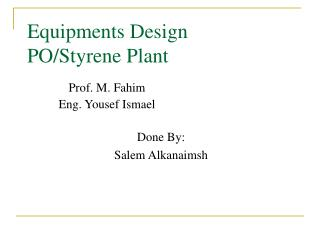DownloadDownload PresentationEquipments Design PO/Styrene Plant

Equipments Design PO/Styrene Plant

Download PresentationEquipments Design PO/Styrene Plant

- - - - - - - - - - - - - - - - - - - - - - - - - - - E N D - - - - - - - - - - - - - - - - - - - - - - - - - - -
Presentation Transcript

1. Equipments DesignPO/Styrene Plant Done By: Salem Alkanaimsh Prof. M. Fahim Eng. Yousef Ismael

2. Agenda • Reactor Design. • Heat Exchangers Design. • Distillation Columns Design. • Pumps Design. • Compressors Design.

3. Reactor Design • Chemical reactors are the heart of chemical processes. • Reactors can be divided into: • Batch reactors. • Continues reactors. • CSTR. • PFTR. • Example: Petroleum Refinery.

4. Reactor Design Finding The rate equation: Design Equation

5. Reactor Design Thickness of Reactor Diameter and length of reactor Where; t: thickness of reactor. P: internal pressure . ri: radius of the vessel . Ej: joint efficiency . S: stress of carbon steal . Cc: corrosion allowance

6. Heat Exchanger Design • Definition. • Service. • Exchanger. • Condenser. • Heater. • Type. • Shell and tube. • Air cooled HX.

7. Shell and Tube HX • Tubes. • Pattern of Tubes. • Shell and nozzle. • Baffles.

8. Shell & Tube Heat Exchanger Design. • Assumptions: • Shell and tube heat exchanger counter flow is used because it is more efficient than the parallel flow. • The value of the overall heat transfer coefficient was assumed based on the fluid assigned in both sides. • The outer, the inner diameter , the length of the tube, and the number of passes were assumed. • For a good design : • The assumed overall heat coefficient has to be equaled to the calculated overall heat transfer coefficient., • The pressure drop in the tube side has to be lower than 1 bar. • The pressure drop in the shell side has to be lower than 1 bar.

9. Shell & Tube Heat Exchanger Design. Log mean Temperature Heat Load Where; T1 is temperature of inlet hot stream. (oC) T2 is the temperature of outlet hot stream. (oC) .t1 is the temperature of inlet cold stream. (oC) .t2 is the temperature of outlet cold stream. (oC).

10. Shell & Tube Heat Exchanger Number of tubes Heat Transfer Area Shell and Bundle diameter Where ; Nt is the number of tubes. K1, n1 are constants. Db is the bundle diameter (mm) Ds is the shell diameter. (mm)

11. Shell & tube heat exchanger Design Tube Side Heat Transfer Coefficient Shell side heat Transfer Coefficient Where is the density of fluid (kg/m3). is the thermal conductivity (W/m.C). is specific heat (kJ/kg.k). Re is the Reynolds number. Pr is the Prandtl number. Nu is the Nusselt number. is the convective heat transfer coefficient (W/m2.C). Where; .pt is the tube pitch (mm). .lB is the baffle spacing (mm). As is the cross flow area (m2) us is the velocity (m/s). de is the equivalent diameter for triangular arrangement (mm). jh is the heat transfer factor hs is the convective heat transfer coefficient (W/m2.C).

12. Shell & Tube Heat Exchanger Design. Overall Heat Transfer coefficient Shell Side Pressure Drop Thickness Tube side pressure Drop Where; D is the shell diameter in m Rj is internal radius in (in). P is the operating pressure in psi S is the working stress (psi). E is the joint efficiency

13. Distillation column • A separation unit based on the difference between a liquid mixture and the vapor formed from it. • It can be subdivided according to: • How complex the unit is: • Simple Distillation. • Flash distillation. • Fractionation. • The internal Design of the column: • Tray Column. • Packing Column.

14. Assumptions: Column Efficiency. Tray spacing. Flooding Percentage. Down Comer Area. Hole area( 0.1 of Active area). Weir height ( 40~100)mm. Hole diameter (10 mm). Plate Thickness (10~30 mm). Turn down Percentage (70%) Good Design: No weeping. Down comer back up is less than half ( plate thickness+ weir height). No entrainment. Calculated percentage flooding equal to the assumed one. Residence time exceeds 3 secs. Distillation Column Design.

15. Distillation Column Design. Actual Number of trays Column Diameter Where; FLV is the vapor-liquid flow factor. is the density in (kg/m3). is the surface tension in (mN/m). uf is the velocity of vapor in (m/s). D is the column diameter (m).

16. Distillation Column Design Provisional Plate design Liquid Flow Pattern

17. Distillation Column Design Checking Weeping Down Comer Back up Residence time

18. Distillation column design Estimating the Thickness Entrainment Cost Number of holes

19. Pump Design • Definition. • Suction Calculations. • Discharge Calculations. • NPSH.

20. Pump Design. Actual Head of pump Water horse Power Efficiency

21. Compressor Design • Definition. • Types: • Centrifugal. • Axial. • Reciprocating. • Compression: • Adiabatic. • Isothermal. • Intercooler stage • Pressure Ratio (PR). Reduce temperature and work required.

22. Compressors Design. Work Efficiency =0.8 Isentropic Compression

23. Thank you All for Paying Attention